# Test: Mid Point Theorem

## 10 Questions MCQ Test Mathematics (Maths) Class 9 | Test: Mid Point Theorem

Description
This mock test of Test: Mid Point Theorem for Class 9 helps you for every Class 9 entrance exam. This contains 10 Multiple Choice Questions for Class 9 Test: Mid Point Theorem (mcq) to study with solutions a complete question bank. The solved questions answers in this Test: Mid Point Theorem quiz give you a good mix of easy questions and tough questions. Class 9 students definitely take this Test: Mid Point Theorem exercise for a better result in the exam. You can find other Test: Mid Point Theorem extra questions, long questions & short questions for Class 9 on EduRev as well by searching above.
QUESTION: 1

### D, E, F are midpoints of sides AB, BC and CA of ΔABC, if ar(ΔABC) = 64 cm2 then, area of ΔBDE is:

Solution:

Bcs (ABC) is a full triangle ABC triangle divide into four equal partsTherefore, 64÷4=16 cm2

QUESTION: 2

### In the adjoining figure, ABCD and PQRC are rectangles, where Q is the midpoint of AC. Then DP is equal to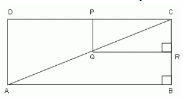Solution:

Given that PQRS and ABCD are rectangle and Q is the mid pt of AC.

Since PQRS and ABCD are rectangle, every angle would be of 90°.

angle QPC = 90°

Now, angle ADP lies corresponding to angle QPC and are equal.

If corresponding angles are equal, then the lines are parallel

Q is the midpoint of AC (Given)

We know that,
line drawn parallel from the midpoint of a side, to other side, bisects the third side.

Hence, P would be the midpoint of DC

Hence, DP = PC

QUESTION: 3

### In triangle ABC, E and F are the mid points of the sides AC and AB respectively. The altitude AP to BC intersects EF at Q. Then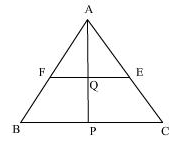Solution:
QUESTION: 4

Figure shows that AD and BF are medians of ABC and BF || DE then CE is equal to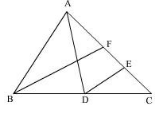Solution:

A median divides the triangle into 2 equal parts. So AD divides the triangle into the triangle ABD & ACD . Further BF divides the triangle into 2 equal parts .
So AF = 1/2 AC
DE bisects it into 2 parts
So EF = 1/2 EC
EF =FC
AF = EC
SO FC =1/2 * 1/2 AC
= 1/4 AC

QUESTION: 5

In ΔABC, D, E and F are respectively mid points of BC, CA and AB. If the lengths of side AB, BC and CA are 7 cm, 8 cm and 9 cm respectively, the perimeter of DEF will be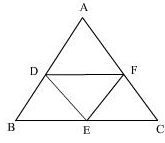Solution:

Since, D,E,F are the midpoints of BC,CA and AB,Thus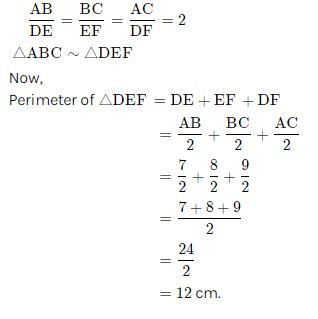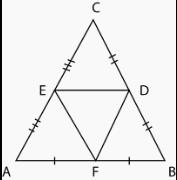QUESTION: 6

If D, E and F are the mid points of the sides BC, CA and AB of an equilateral triangle ABC, then triangle DEF is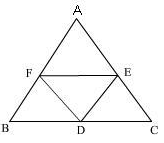Solution:
QUESTION: 7

The quadrilateral formed by joining the midpoints of the pairs of adjacent sides of a rectangle is a

Solution:
QUESTION: 8

ABCD is a parallelogram in which P, Q, R and S are the mid points of sides AB, BC CD and DA respectively. Then,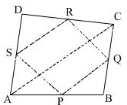Solution:
QUESTION: 9

Find the area of a trapezium whose parallel sides are 24 cm and 20 cm and the distance between them is 15 cm.

Solution:
QUESTION: 10

In the adjoining figure, the side AC of ABC is produced to E such that CE = 1/2 AC. If D is the midpoint of BC and ED produced meets AB at F, and CP, DQ are drawn parallel to BA, then FD is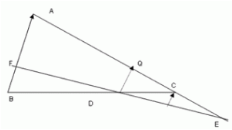Solution:

Given,
ABC is a triangle.
D is midpoint of BC and DQ is drawn parallel to BA.
Then, Q is midpoint of AC.
∴ AQ = DC
∴ FA parallel to DQ||PC.
AQC, is a transversal so, AQ = QC and FDP also a transveral on them.
∴ FD = DP .......(1) [ intercept theorem]
EC = 1/2 AC = QC
Now, triangle EQD, here C is midpoint of EQ and CP which is parallel to DQ.
And, P is midpoint of DE.
DP = PE..........(2)
Therefore, From (1) and (2)
FD = DP = PE
∴ FD = 1/3 FE